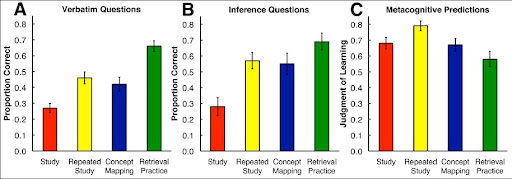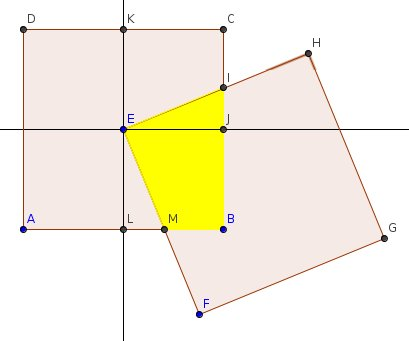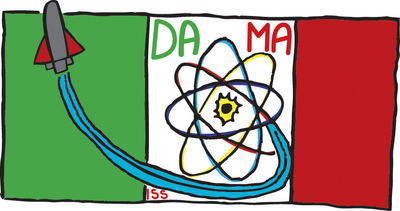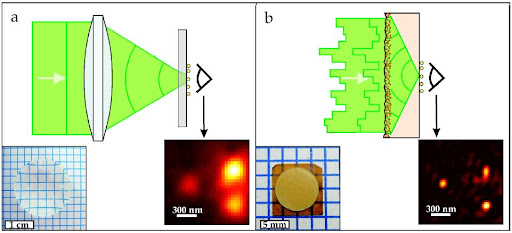### The Nice model of Solar System

When I see the previous video (via Keplero) I immediatly thought to the Nice model, a simulation model about our Solar System developed by Rodney Gomes, Harold F. Levison, Alessandro Morbidelli, Kleomenis Tsiganis in three papers published on Nature vol.235
First of all a little resume about Solar System formation thoery. Following Kant-Laplace model, our Solar System was born from massive and dense clouds of molecular hydrogen—giant molecular clouds. In this nebula occurs planets formation. In particular theory supposed that giant planets formed on circular and coplanar orbits(3, 5). In this picture, all planets aresubstantially formed in the same position of the actual System. The Nice model suggests that all Solar System objects are formed in a different position and a perturbation in orbits forced the actuall more stable orbits.
The original model's core was developed by Gomes, Morbidelli and Levison in 2004(7)
We study planetary migration in a gas-free disk of planetesimals. In the case of our Solar System we show that Neptune could have had either a damped migration, limited to a few AUs, or a forced migration up to the disk’s edge, depending on the disk's mass density. We also study the possibility of runaway migration of isolated planets in very massive disk, which might be relevant for extra-solar systems. We investigate the problem of the mass depletion of the Kuiper belt in the light of planetary migration and conclude that the belt lost its pristine mass well before that Neptune reached its current position. Therefore, Neptune effectively hit the outer edge of the proto-planetary disk. We also investigate the dynamics of massive planetary embryos embedded in the planetesimal disk. We conclude that the elimination of Earth-mass or Mars-mass embryos originally placed outside the initial location of Neptune also requires the existence of a disk edge near 30AU.
In this first paper there's an analytic toy model for migration process. First of all they calculate the variation in time of the semi-major axis $a_P$ of the planet:
$\frac{\text{d} a_P}{\text{d} t} = \frac{k}{2 \pi} \frac{M(t)}{M_P} \frac{1}{\sqrt{a_P}}$
where $M(t)$ is the amount of material in orbits that cross the orbit of the planet, $M_P$ the mass of the planet, $k$ a parameter of the distribution of those orbits.
The evolution of $M(t)$ is described by the following equation:
$\dot M (t) = -\frac{M(t)}{\tau} + 2 \pi a_P |\dot a_P| \sigma (a_P)$
where $\tau$ is decay time of planetesimals, $\sigma$ the surface density of not yet scattered planetesimals, $\dot a_P$ the planetary migration rate, $\dot M (t)$ the decay of the planetesimal population due to the planetesimal's finite dynamical lifetime.
Sobstituting the first equation in the second, it can obtain:
$\dot M (t) = \left ( \frac{1}{\tau} + |k| \sqrt{a_P} \frac{\sigma(a_P)}{M_P} \right ) M(t)$
And the solution of this equation is given by
$M(t) = M(0) \text{e}^{\alpha t}$
where
$\alpha = \frac{1}{\tau} + |k| \sqrt{a_P} \frac{\sigma(a_P)}{M_P}$
that is time independent.
We can have two different situations: $\alpha$ negative, $\alpha$ positive.
In the first case the migration speed is too low to compensate the loss of planetesimals: the migration mode is called damped migration.
In the second case $M(t)$ grows exponentially, and migration, called forced migration, is self-sustained.
After the giant planets were formed and the circumsolar gaseous nebula was dissipated, the Solar System was composed of the Sun, the planets and a debris disk of small planetesimals.
Planets' migration so is caused by the change of angular momentum during the scattering with planetesimals.
Numerical simulations(4) show that Jupiter was forced to move inward, while Saturn, Uranus and Neptune drifted outward.
An example of the output produced by Nice simulations is the following plot:

### Don't panic! It's Towel Day

Wikipedia writes:
Towel Day is celebrated every 25 May as a tribute by fans of the late author Douglas Adams. On this day, fans carry a towel with them to demonstrate their love for the books and the author, as referenced in Adams's The Hitchhiker's Guide to the Galaxy. The commemoration was first held in 2001, two weeks after Adams's death on 11 May 2001.
I forget my towel, so I post this Linus image: don't panic!I search on Scholar The Hitchhiker's Guide to the Galaxy and I find some references
The Hitchhiker's Guide To Teaching Legal Research To The Google Generation (pdf)
A guide to the Guide by Cohen-Rose and Christiansen (Google Books preview)
Equation-of-Motion Coupled-Cluster Methods for Open-Shell and Electronically Excited Species: The Hitchhiker's Guide to Fock Spacez by Anna I. Krylov
And there's also a ref. in Penrose's book The Road to Reality: A Complete Guide to the Laws of the Universe
I conclude with a video from the official site of Towel Day

### Retrieval learning practice

Jeffrey Karpicke and Janell Blunt published on Science express a report about their research on retrieval practice in learning.
Karpicke studied the subject just in 2008 with Roediger III in The critical importance of retrieval for learning. Here and in the successful paper he confrounts retrieval with elaborative studying. Just in the 2008 paper he writes
repeated testing produced a large positive effect
But what is retrieval?
I found on Purdue the following definition:
Retrieval is a process of recalling what we have in memory.
So retrieval practice is the practice used by students to recall informations. In this vision I think that retrieval and elaborative studying are not so different in principle in the use of our brain, but, according to Karpicke and Blunt's results, retrieval is probably more useful for exams and elaborative is more useful if you want obtain more persistence results.
Karpicke and Blunt performed two experiments. In the first test 80 Purdue University undergraduate students studied on Sea Otters. Students are divided in 4 groups: study, repeated study, concept mapping, retrieval practice.
In data elaboration researchers pay attention in different learning activities: they can depend on the strucuture of the materials.
The conclusions:
Research on retrieval practice suggests a view of how the human mind works that differs from everyday intuitions. Retrieval is not merely a read out of the knowledge stored in one's mind – the act of reconstructing knowledge itself enhances learning. This dynamic perspective on the human mind can pave the way for the design of new educational activities based on consideration of retrieval processes.
are supported by data, summarized in the following istograms:### Square deal: a solution

On Futility Closet, Greg Ross proposed an interesting puzzle that i reproduce in the following picture, using GeoGebra:The result of the quest (calculate yellow area, or $A_{EIBM}$) is 4 inch2. Why?
First of all we must proof the congruence between $T_{AEIBM}$ and $T_{EKCJ}$, who is a square's quarter, and so $A_{EKCJ} = 4$.

### Italians in spaceDAMA mission's logo is designed by an italian middle school student, and mission name is choiced by another young pupil using the first two letters ofthe words dark matter.
The mission man is Roberto Vittori: he'll use AMS-02. Using this device, he will detect dark matter and antimatter by observing the cosmic rays, helping us hopefully to better understand the origin and structure of the Universe.
Roberto will act also some experiments in life science, technology, medicine, biology and material science.From ESA's pdf I extract some quotes about AMS:
AMS is embarking on a mission to explore distant and
uncharted realms of our Universe, where answers to some longstanding questions in particle physics and cosmology may be revealed and unexpected phenomena may be discovered.
Mission's keywords are cosmic rays, antimatter, dark matter, dark energy.
Cosmic rays are composed by radiation and stable particles from stars, but when they arrive to our planet, they interact with atmosphere. So it is need to use a detector in space, and astronomers and particle physicists are eagerly awaiting the data.
Antimatter is, in a very simple picture, same to the matter but with opposed charge, and is more unstable.
AMS will search for antimatter to the edge of the observable universe.
Finally, according to theories and indirect observations, we know to... unknow 95% of the universe! Experiment was projected by prof.Ting.
Prof. Ting's experiment will increase the sensitivity of the search by between a thousand and a million times, revealing a totally different domain by either finding the neutralino or revealing something else. AMS-02 might also detect a new, exotic form of matter predicted by scientists: a very heavy elementary particle dubbed 'strangelet'.Another important event is the presence on International Space Station of two italians, Roberto Vittori, who arrived with STS-134 mission. And on ISS there is Paolo Nespoli, who publishes his spectacular photos on flickr.
A great event for all Italian poeple!

(via ESA)

### Press Release: Scattering lens yields unprecedented sharp images

Jacopo Bertolotti is a wikipedian friend. Some weeks ago he sent me his last paper, Scattering lens resolves sub-100 nm structures with visible light about a research work that he relised in Netherlands at Complex Photonic Systems (Institute for Nanotechnology, University of Twente). It's an interesting paper about the construction of the first lens that provides a resolution in the nanometer regime at visiblewavelengths (I read in the abstract).
In this moment I read the paper, that it is published on Phys. Rev. Lett. 106 on the 13rd May 2011, the black day of Blogger.
So, before a post in which I examine in details the paper, I decide to publish the official press release of the team:
It is generally believed that disorder always degrade the sharpness of optical images. Now scientists of the MESA+ Institute at the University of Twente, University of Florence and the FOM Institute AMOLF have shown that a scattering and disordered layer in conjunction with a high refractive index material can be used as an imaging device with a sub-100 nm resolution thereby beating the most expensive microscope objectives. The robustness of this scattering lens against distortion and aberrations, together with the ease of manufacturing and its very high resolution are highly favorable features to improve the performance of a wide range of cutting-edge microscopy techniques. The results are being published this Friday in the leading journal Physical Review Letters and are highlighted as an Editors' suggestion.

Even the most expensive microscope objectives offer only a limited resolution. This restriction is due to the wave nature of light that force any focus to be larger than half the wavelength of light (the diffraction limit). This theoretical limit is usually impossible to reach due to practical problems like aberrations that cause focal distortion. Paradoxically a completely disordered layer naturally creates very small and intense light spots when illuminated by a laser. The price to pay is that these spots, which are known as speckle, are arranged in a dense and random pattern making them useless for imaging purposes.
The new scattering lens developed by the scientists, uses light scattering to couple light efficiently into a high refractive index material. By a fine control over the light that illuminates the disordered layer they can concentrate the speckle spots in the same place, effectively creating a single very small focus. Taking advantage of what is known as the "memory effect" the scientists were able to scan this nano-sized focus in the object plane of the lens. They then placed small gold nano particles in the object plane and used the scattering lens to resolve the particles with a sub-100 nm resolution.
The combination of a high-index scattering material with the complete control over the illumination provides the first lens that is able to resolve nano-structures with visible light. The ability of this scattering lens to create small and scannable focuses makes it a favorable tool to improve the performance of all the imaging methods that require accurate focusing.Comparison of light focusing with a conventional lens and a scattering lens. (a) A plane light wave sent through a normal lens forms a focus. The focal size is determined by the range of angles in the converging beam as and by the refractive index of the medium that the light is propagating in. The microscope image shows a collection of gold spheres as imaged with a commercial high quality oil immersion microscope objective. Inset on left is a photo of an ordinary lens. (b) The scientists send a shaped wave through a scattering layer on top of a high refractive index material. The wave front is carefully shaped so that, after traveling through the layer, it forms a perfectly spherical, converging wave front. The large range of angles contributing to the converging beam, combined with the high refractive index, give rise to a nanometer-sized focal spot. The microscope image shows the same collection of gold spheres as in (a) imaged with the scattering lens. Inset on left is a photo of the lens with the scattering layer on top.

If you want read paper, but don't have the subscription to PRL, you can read arXiv preprint.

### Ratios of Iodine-131 to Cesium-137 at the Fukushima reactors

A few days ago it was uploaded on arXiv a preprint about radiactions from Fukushima reactors, Deciphering the measured ratios of Iodine-131 to Cesium-137 at the Fukushima reactors, by T. Matsui (University of Tokyo).
In the preprint, Matsui propose some simple theoretical calculations to evaluate the situation of TEPCO's reactors.
The physical basis is the radioactive decay,
$N(t) = N_0 e^{-\lambda t}$
where $N_0$ is the number of nucleus at time $t_0$ (in the beginning), $\lambda$ is the decay rate (inverse of life time $\tau$), $N (t)$ is the number of nucleus at time $t$.
To calculate the equation we start from the experimental law,
$\frac{\text d N (t)}{\text d t} = - N(t) \lambda$
For his calculation, Matsui used the following differential equation
$\frac{\text d N_I (t)}{\text d t} = f_I N_0 \theta (t;t_i, t_f) - \lambda_I N_I$
where $\theta (t;t_i, t_f) = 1$ for $t_i < t < t_f$ and $\theta (t;t_i, t_f) = 0$ otherwise, $N_0$ is the number of fission in time, $f_I$ is the fraction of I-131 produced in every fission, $\lambda_I$ the decay rate of I-131. Boundary conditions are: nuclear reactor had been in operation from $t_i$ to $t_f$; $N_I (t_i) = 0$. After integration, and introducing the condition $\lambda_I (t_f - t_i) \gg 1$ (it means that the working time of the reactor is much longer than the half-life of I-131, that is 8 days), Matsui found: